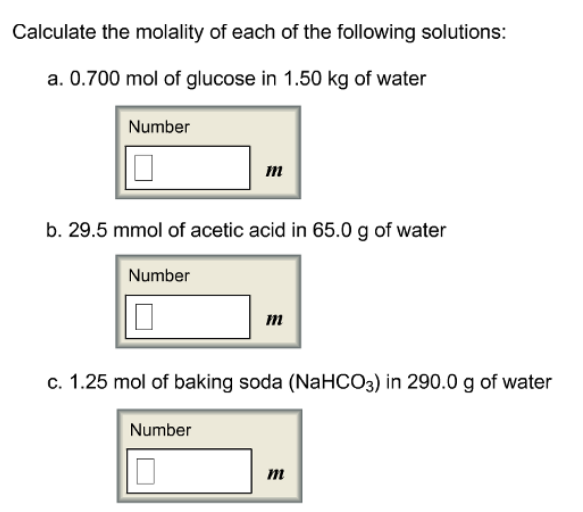# Problem: Calculate the molality of each of the following solutions:a. 0.700 mol of glucose in 1.50 kg of waterb. 29.5 mmol of acetic acid in 65.0 g of waterc. 1.25 mol of baking soda (NaHCO3) in 290.0 g of water

###### FREE Expert Solution
92% (196 ratings)###### Problem Details

Calculate the molality of each of the following solutions:

a. 0.700 mol of glucose in 1.50 kg of water

b. 29.5 mmol of acetic acid in 65.0 g of water

c. 1.25 mol of baking soda (NaHCO3) in 290.0 g of water